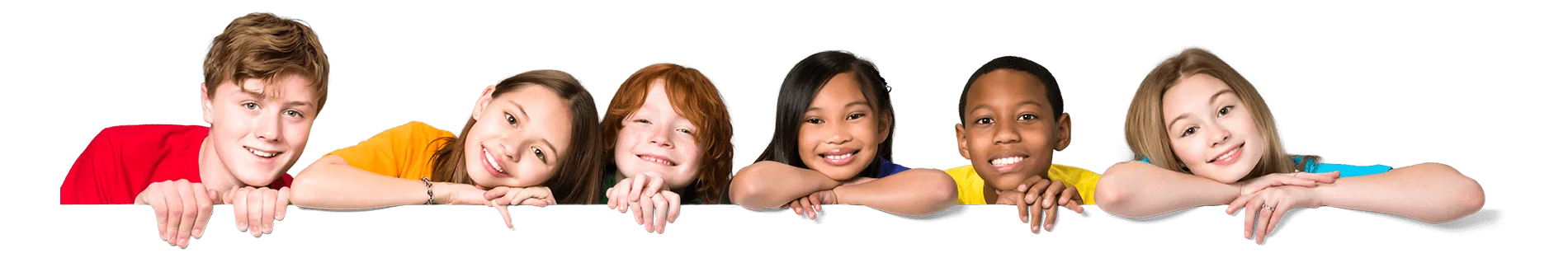Latest Results:

# 11plus Letter Sequences sample papers

Solve this Question

#### Find the missing letters that completes the following sequence. The alphabet has been given to help you.A B C D E F G H I J K L M N O P Q R S T U V W X Y ZA,C,E,G,____,____

 1. I,K 2. K,I 3. L,N 4. N,L

Solve this Question

#### Find the missing letters that completes the following sequence. The alphabet has been given to help you.A B C D E F G H I J K L M N O P Q R S T U V W X Y ZC, V, D, X, ___, ___

 1. E, J 2. J, L 3. E, Z 4. Z, E

Solve this Question

#### Find the missing letters that completes the following sequence. The alphabet has been given to help you.A B C D E F G H I J K L M N O P Q R S T U V W X Y ZAC, BD, EG, FH, ___, ___

 1. IK, JL 2. IL, JK 3. SU, TV 4. ST, UV

Solve this Question

#### Find the missing letters that completes the following sequence. The alphabet has been given to help you.A B C D E F G H I J K L M N O P Q R S T U V W X Y ZC,F,I,L,__, ___

 1. O,Q 2. P,R 3. O,R 4. P,Q